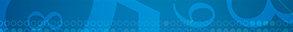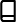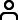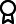Journal metrics
See full report
Acceptance rate20%
Submission to final decision52 days
Acceptance to publication28 days
CiteScore1.600
Journal Citation Indicator0.330
Impact Factor-

### Indexing news

International Journal of Mathematics and Mathematical Sciences has recently been accepted into Web of Science.

####Journal profile

International Journal of Mathematics and Mathematical Sciences publishes research across all fields of mathematics and mathematical sciences, such as pure and applied mathematics, mathematical physics, probability and mathematical statistics.

####Editor spotlight

International Journal of Mathematics and Mathematical Sciences maintains an Editorial Board of practicing researchers from around the world, to ensure manuscripts are handled by editors who are experts in the field of study.

####Special Issues

Do you think there is an emerging area of research that really needs to be highlighted? Or an existing research area that has been overlooked or would benefit from deeper investigation? Raise the profile of a research area by leading a Special Issue.

## Latest Articles

More articles
Research Article

### Extended Newton-type Method for Nonsmooth Generalized Equation under -point-based Approximation

Let and be Banach spaces and . Let be a single valued function which is nonsmooth. Suppose that is a set-valued mapping which has closed graph. In the present paper, we study the extended Newton-type method for solving the nonsmooth generalized equation and analyze its semilocal and local convergence under the conditions that is Lipschitz-like and admits a certain type of approximation which generalizes the concept of point-based approximation so-called -point-based approximation. Applications of -point-based approximation are provided for smooth functions in the cases and as well as for normal maps. In particular, when and the derivative of , denoted , is -Hölder continuous, we have shown that admits -point-based approximation for while admits -point-based approximation for , when and the second derivative of , denoted , is -Hölder. Moreover, we have constructed an -point-based approximation for the normal maps when has an -point-based approximation. Finally, a numerical experiment is provided to validate the theoretical result of this study.

Research Article

### Generalized Fubini Apostol-Type Polynomials and Probabilistic Applications

The paper aims to introduce and investigate a new class of generalized Fubini-type polynomials. The generating functions, special cases, and properties are introduced. Using the generating functions, various interesting identities, and relations are derived. Also, special polynomials are obtained from the general class of polynomials. Finally, probabilistic applications and some probabilistic properties are obtained.

Research Article

### Mathematical Model Analysis on the Diffusion of Violence

Recently, violence has been a very common and serious public health problem in the world. In this new mathematical modeling tactic study, we formulated and examined the firsthand violence mathematical model with five distinct classes of the human population (susceptible, violence-exposed, violence, negotiated, and reconciled). The model takes into account the diffusion of violence and infection. The violence-free and violence-dominance model equilibrium points are calculated, and their local and global stabilities are analyzed. The model threshold values are obtained. As a result of the model analysis, the violence diffusion is under control if the basic reproduction number is less than unity, and it diffuses through the community if this number exceeds unity. Besides, the sensitivity analysis of the parameter values of the basic reproduction number is demonstrated. We have applied the MATLAB ode45 solver to illustrate the numerical results of the model. Finally, from analytical and numerical solutions, we obtain jointly equivalent and consistent results.

Research Article

### The Bifurcation Curves of a Category of Dirichlet Boundary Value Problems

We study the Dirichlet boundary value problem generally and develop a schema for determining the relationship between the values of its parameters and the number of positive solutions. Then, we focus our attention on the special cases when and , respectively. We prove first that all positive solutions of the first problem are less than or equal to , obtain more specific lower and upper bounds for these solutions, and compute a curve in the -plane with accuracy up to , below which the first problem has a unique positive solution and above which it has exactly three positive solutions. For the second problem, we determine its number of positive solutions and find a formula for the value of that separates the regions of , in which the problem has different numbers of solutions. We also computed the graphs for some special cases of the second problem, and the results are consistent with the existing results. Our code in Mathematica is available upon request.

Research Article

### Harmonic Mixture Fréchet Distribution: Properties and Applications to Lifetime Data

In this study, we propose a four-parameter probability distribution called the harmonic mixture Fréchet. Some useful expansions and statistical properties such as moments, incomplete moments, quantile functions, entropy, mean deviation, median deviation, mean residual life, moment-generating function, and stress-strength reliability are presented. Estimators for the parameters of the harmonic mixture Fréchet distribution are derived using the estimation techniques such as the maximum-likelihood estimation, the ordinary least-squares estimation, the weighted least-squares estimation, the Cramér–von Mises estimation, and the Anderson–Darling estimation. A simulation study was conducted to assess the biases and mean square errors of the estimators. The new distribution was applied to three-lifetime datasets and compared with the classical Fréchet distribution and eight (8) other extensions of the Fréchet distribution.

Research Article

### The Metric Chromatic Number of Zero Divisor Graph of a Ring

Let be a nontrivial connected graph, be a vertex colouring of , and be the colouring classes that resulted, where . A metric colour code for a vertex a of a graph is , where is the minimum distance between vertex and vertex in . If , for any adjacent vertices and of , then is called a metric colouring of as well as the smallest number satisfies this definition which is said to be the metric chromatic number of a graph and symbolized . In this work, we investigated a metric colouring of a graph and found the metric chromatic number of this graph, where is the zero-divisor graph of ring .Journal metrics
See full report
Acceptance rate20%
Submission to final decision52 days
Acceptance to publication28 days
CiteScore1.600
Journal Citation Indicator0.330
Impact Factor-Author guidelinesEditorial boardDatabases and indexingAuthor guidelinesEditorial boardDatabases and indexing

Article of the Year Award: Outstanding research contributions of 2021, as selected by our Chief Editors. Read the winning articles.